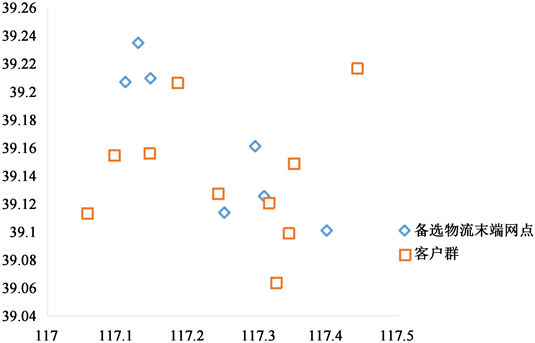#### 期刊菜单

Research on the Location of Logistics Terminal Outlets Based on Comprehensive Satisfaction
DOI: 10.12677/MSE.2022.114078, PDF, HTML, XML, 下载: 22  浏览: 41

Abstract: After the continuous development of modern logistics in recent years, it has become an important pillar and basic industry of national economic development, and its development degree has also become one of the important indicators to measure the degree of modernization and comprehensive national strength of a country. The logistics terminal network is an important logistics node in the modern logistics system, and its reasonable location plays a very important role in the healthy and rapid development of modern logistics in China. In this paper, the gray prediction model is first used to do curve fit of the historical data of the logistics company’s volume through the spssau software, and the future demand of customers in 2022 is reasonably predicted. Secondly, on the basis of the existing logistics terminal network location model, this paper establishes a self-pickup logistics terminal network location model, and considers the influence of customer satisfaction and enterprise satisfaction on the location problem of logistics terminal network in the model, constructs a multi-objective location model of logistics terminal network based on comprehensive satisfaction, and converts it into a single-objective model by linear weighted summation. Finally, through software solution, the site selection scheme under different weights is obtained. Through the test, the method meets the actual requirements and development of logistics terminal network site selection.

1. 引言

2. GM(1,1)模型设置

GM(1,1)模型表示一阶的、一个变量的微分方程模型，是最常用的灰色模型之一。在物流中，运输量等表征量一次累加生成序列为严格的单增序列，可以使用指数函数进行拟合。目前使用最广泛的灰色预测模型是关于数据预测的一个变量、一阶微分的GM(1,1)模型。它是基于随机的原始时间序列，经按照时间累加后形成的新的时间序列呈现的规律可用一阶线性微分方程的解来逼近，因此可以采用GM(1,1)模型进行物流需求量的预测。

GM(1,1)模型的一阶微分方程为：

${X}^{\left(0\right)}=\left\{{x}^{\left(0\right)}\left(k\right),k=1,2,\cdots ,n\right\}$

${X}^{\left(1\right)}=\left\{{x}^{\left(1\right)}\left(k\right),k=1,2,\cdots ,n\right\}$

${x}^{\left(1\right)}\left(k\right)=\underset{i=0}{\overset{k}{\sum }}x\left(i\right)\text{\hspace{0.17em}}\text{\hspace{0.17em}}\left(k=1,2,\cdots ,n\right)$

$Y={\left[{x}^{\left(0\right)}\left(2\right),{x}^{\left(0\right)}\left(3\right),\cdots ,{x}^{\left(0\right)}\left(n\right)\right]}^{\text{T}}$

$B=\left[\begin{array}{cc}-\frac{1}{2}\left({x}^{\left(1\right)}\left(1\right)+{x}^{\left(1\right)}\left(2\right)\right)& 1\\ -\frac{1}{2}\left({x}^{\left(1\right)}\left(3\right)+{x}^{\left(1\right)}\left(3\right)\right)& 1\\ ⋮& ⋮\\ -\frac{1}{2}\left({x}^{\left(1\right)}\left(n-1\right)+{x}^{\left(1\right)}\left(n\right)\right)& 1\end{array}\right]$

$Y=B\left[\begin{array}{c}a\\ u\end{array}\right]$

GM(1,1)模型的离散解还原后得到：

${\stackrel{^}{x}}^{\left(0\right)}\left(k\right)={\stackrel{^}{x}}^{\left(1\right)}\left(k\right)-{\stackrel{^}{x}}^{\left(1\right)}\left(k-1\right)$

3. 基于综合满意度的物流末端网点选址模型

3.1. 模型假设

1) 将客户根据地理位置划分为客户群；

2) 不考虑网点之间的竞争关系，且每个客户群有且仅有一个网点为其配送；

3) 客户群被末端网点全覆盖；

4) 每个客户群需求量在一定时间内稳定不变。

3.2. 符号说明

${N}_{m}=\left(1,2,\cdots ,m\right)$ 是所有备选物流末端网点的集合。m为备选物流末端网点的个数。

${N}_{n}=\left(1,2,\cdots ,n\right)$ 是客户群的集合。n为客户群的个数。

xi，yij是0~1变量。其中， $i\in {N}_{m}$$j\in {N}_{n}$

dij是备选物流末端网点i到客户群j的距离。

da是备选物流末端网点i到客户群j的最大距离。

dk是备选物流末端网点i到客户群j的理想距离。

gi是备选物流末端网点i的建设费用。

r是物流末端网点的人均工资。

Cm是企业可接受的最大成本。

Qj是客户群j的货物需求量。

3.3. 问题分析

1) 客户满意度

$f\left({d}_{ij}\right)=\left\{\begin{array}{l}1\text{\hspace{0.17em}}\text{\hspace{0.17em}}\text{\hspace{0.17em}}\text{\hspace{0.17em}}\text{\hspace{0.17em}}\text{\hspace{0.17em}}\text{\hspace{0.17em}}\text{\hspace{0.17em}}\text{\hspace{0.17em}}\text{\hspace{0.17em}}\text{\hspace{0.17em}}\text{\hspace{0.17em}}\text{\hspace{0.17em}}\text{\hspace{0.17em}}\text{\hspace{0.17em}}\text{\hspace{0.17em}}\text{\hspace{0.17em}}\text{\hspace{0.17em}}\text{\hspace{0.17em}}\text{\hspace{0.17em}}\text{\hspace{0.17em}}\text{\hspace{0.17em}}\text{\hspace{0.17em}}\text{\hspace{0.17em}}\text{\hspace{0.17em}}\text{\hspace{0.17em}}{d}_{ij}\le {d}_{k}\\ 1-{\left(\frac{{d}_{ij}-{d}_{k}}{{d}_{a}-{d}_{k}}\right)}^{\beta }\text{\hspace{0.17em}}\text{\hspace{0.17em}}\text{\hspace{0.17em}}\text{\hspace{0.17em}}{d}_{k}<{d}_{ij}<{d}_{a}\\ 0\text{\hspace{0.17em}}\text{\hspace{0.17em}}\text{\hspace{0.17em}}\text{\hspace{0.17em}}\text{\hspace{0.17em}}\text{\hspace{0.17em}}\text{\hspace{0.17em}}\text{\hspace{0.17em}}\text{\hspace{0.17em}}\text{\hspace{0.17em}}\text{\hspace{0.17em}}\text{\hspace{0.17em}}\text{\hspace{0.17em}}\text{\hspace{0.17em}}\text{\hspace{0.17em}}\text{\hspace{0.17em}}\text{\hspace{0.17em}}\text{\hspace{0.17em}}\text{\hspace{0.17em}}\text{\hspace{0.17em}}\text{\hspace{0.17em}}\text{\hspace{0.17em}}\text{\hspace{0.17em}}\text{\hspace{0.17em}}\text{\hspace{0.17em}}{d}_{ij}\ge {d}_{k}\end{array}$ (2-1)

${\omega }_{j}=\frac{{z}_{j}}{{\sum }_{j\in {N}_{n}}{y}_{ij}{z}_{j}}$ (2-2)

${k}_{i}={\sum }_{j\in {N}_{n}}{y}_{ij}{\omega }_{j}f\left({d}_{ij}\right)$ (2-3)

${F}_{1}=\frac{{\sum }_{i\in {N}_{m}}{x}_{i}{k}_{i}}{{\sum }_{i\in {N}_{m}}{x}_{i}}$ (2-4)

2) 企业满足度

${C}_{1}={\sum }_{i\in {N}_{m}}{g}_{i}{x}_{i}$ (2-5)

${C}_{2}={\sum }_{i\in {N}_{m}}{x}_{i}\left[round\left(\frac{{\sum }_{j\in {N}_{n}}{Q}_{j}{y}_{ij}}{K}\right)r\right]$ (2-6)

$c={\sum }_{i\in {N}_{m}}{g}_{i}{x}_{i}+{\sum }_{i\in {N}_{m}}{x}_{i}\left[round\left(\frac{{\sum }_{j\in {N}_{n}}{Q}_{j}{y}_{ij}}{K}\right)r\right]$ (2-7)

${F}_{2}=1-\frac{c}{{c}_{m}}$ (2-8)

3.4. 模型构建

$\mathrm{max}{F}_{1}=\frac{{\sum }_{i=1}^{m}{\sum }_{j=1}^{n}{x}_{i}{y}_{ij}{\omega }_{j}f\left({d}_{ij}\right)}{{\sum }_{i=1}^{m}{x}_{i}}$ (2-9)

s.t.

$\mathrm{max}{F}_{2}=1-\frac{c}{{c}_{m}}$ (2-10)

${\sum }_{j\in {N}_{n}}{y}_{ij}=1$ (2-11)

${d}_{ij}<{d}_{m}$ (2-12)

$1<{\sum }_{i\in {N}_{m}}{x}_{i} (2-13)

${\sum }_{j\in {N}_{n}}{Q}_{j}{y}_{ij}<{F}_{i}$ (2-14)

${x}_{i}>{y}_{ij}$ (2-15)

0~1变量：

${x}_{i},{y}_{ij}\in \left\{0,1\right\}$ (2-16)

4. 基于综合满意度的物流末端网点选址模型求解

4.1. 多目标模型求解

$\mathrm{max}F={w}_{1}{F}_{1}+{w}_{2}{F}_{2}$

w1、w2为目标函数重客户满意度和企业满足度的权重。在上式中，F为客户满意度和企业满足度二者的综合满足度目标函数，而且 ${w}_{1},{w}_{2}\ge 0$${w}_{\text{1}}+{w}_{\text{2}}=\text{1}$

4.2. 免疫优化算法设计

Step 1：分析问题，可知物流末端网点选址模型的解是0-1变量，故可以采用二进制的编码形式。

Step 2：产生初始抗体群，根据步骤1规定的染色体编码解码规则随机产生N+m个抗体。其中N为种群规模，m为记忆库中抗体数量。

Step 3：将客户群按照距离最近原则选择物流末端网点，根据现实情况在物流末端网点都在客户群可接受的距离范围内时，客户只会选择距离自己最近的物流末端网点，而不会根据模型设定去选择物流末端网点，所以在求解模型时，直接安排客户选择距离自己最近的物流末端网点，才是最合理和符合现实情况。

Step 4：对N + m个抗体进行评价，个体期望繁殖率为标准来评价抗体的优劣程度。

Step 5：进行降序排列形成父代群体，取前N个个体为父代群；根据精英策略保留前m个抗体存入库。

Step 6：判断目标函数是否达到最优值或者算法运行是否达到迭代次数，若满足上面条件则结束，否则继续操作。

Step 7：免疫操作(对父代群执行)：

1) 选择，根据预先设定的选择概率进行选择(轮盘赌法)；

2) 交叉，根据预先设定的交叉概率进行单点交叉；

3) 变异，根据预先设定的变异概率进行随机变异。

Step 8：根据Step 7中进行免疫操作产生的N个新抗体和保留在库中的m个抗体，组成新的N + m个抗体种群；然后转去执行Step 4，直到循环结束。

5. 案例分析Table 1. Alternative logistics terminals and fixed costsTable 2. Customer base in the past 3 years of cargo demandTable 3. Forecast of demand for goods for all customer segmentsFigure 1. Alternative logistics terminal network and customer group coordinate mapTable 4. Site selection scheme under different weights

1) 在满足客户服务的条件下，追求使用较少的成本投入，则选择1，2；

2) 将客户满意度放在第一位，把客户满意度的权重调整到较大且满足成本的情况下，可以选择5，6；

3) 均衡的选址策略，同时考虑客户满意度和企业满足度，并且二者同等重要，可以选择3，4。

6. 结论

  张戎, 王镇豪. 城市配送末端节点布局双层规划模型及算法[J]. 同济大学学报(自然科学版), 2012, 40(7): 1035-1040.  李丁, 黄远新, 田红英. 虚拟环境下3PL企业实施协同配送的仿真研究[J]. 物流技术, 2009, 28(7): 117-120.  Tang, X., Lehuédé, F. and Péton, O. (2016) Location of Distribution Centers in a Multi-Period Collaborative Distribution Network. Electronic Notes in Discrete Mathematics, 52, 293-300. https://doi.org/10.1016/j.endm.2016.03.039  Dan, Z.G., Yu, S.C., Zhen, L., et al. (2016) Multi-Period Distribution Center Location and Scale Decision in Supply Chain Network. Computers & Industrial Engineering, 101, 216-226. https://doi.org/10.1016/j.cie.2016.09.001  He, Y.D., Wang, X., Lin, Y., et al. (2017) Sustainable Decision Making for Joint Distribution Center Location Choice. Transportation Research Part D: Transport and Environment, 55, 202-216. https://doi.org/10.1016/j.trd.2017.07.001  郭鹏, 周洋, 周峰, 等. 城区物流配送中心选址优化系统设计与实现[J]. 河北科技大学学报, 2017, 38(1): 19-25.  魏延青, 庆徐, 荣亚军. 基于救灾物资满意度的最大覆盖选址问题[J]. 物流工程与管理, 2013, 35(1): 50-53.  Hua, X., Hu, X. and Yuan, W. (2016) Research Optimization on Logistics Distribution Center Location Based on Adaptive Particle Swarm Algorithm. Optik: International Journal for Light and Electron Optics, 127, 8443-8450. https://doi.org/10.1016/j.ijleo.2016.06.032